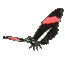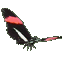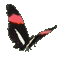Math Help     Speed = Slope     Line Graphs Game Tips:

- The average Speed from a point P to a point Q on a Time-Distance graph or grid
is calculated as the Speed = (change in Distance)/(change in Time) = slope PQ.

- The Speed from P(1, 80) to Q(3,120) is calculated as (120-80)/(3-1) = 40/2 = 20 km/h.

- The slope (rate or speed) from a point P(-2,-3) to a point Q(1,4) on an x-y graph
is calculated as the (rise)/(run) = (4-(-3))/(1-(-2)) = (7)/(3).

- Your Game Score is reduced by the number of butterfly hits.

- To slow the game speed repeat tap/click on the word Slider.
- To increase the game speed repeat tap/click on the word Math.
- Speed can also be adjusted with a keyboard's - and + keys.

- Refresh/Reload the web page to restart the game.